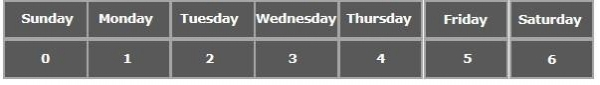# Can we have integers as elements of an enum in Java?

Enumeration (enum) in Java is a datatype which stores a set of constant values. You can use enumerations to store fixed values such as days in a week, months in a year etc.

You can define an enumeration using the keyword enum followed by the name of the enumeration as −

enum Days {
SUNDAY, MONDAY, TUESDAY, WEDNESDAY, THURSDAY, FRIDAY, SATURDAY
}

Just like arrays, the elements/constants in an enumeration are identified using numbers starting from 0 in the above example the days are identified using numbers as shown in the following illustration −## Integers as elements of an enum

No, we can have only strings as elements in an enumeration. Using strings in it, generates a compile time error.

## Example

In the following java example, we are trying to have 3 integer values as elements of an enum.

enum EnumTest {
10024, 5336, 9987
}

## Output

EnumTest.java:1: error: <identifier> expected
enum EnumTest {
^
EnumTest.java:2: error: ',', '}', or ';' expected
10024, 5336, 9987
^
EnumTest.java:2: error: '}' expected
10024, 5336, 9987
^
3 errors

Instead you can have integer values to the elements of an enum.

## Example

enum StudentMarks {
//Constants with values
Krishna(86), Katyayani(75), Bhanu(89), Bhargav(70), Lata(90);
//Instance variable
private int marks;
//Constructor to initialize the instance variable
StudentMarks(int marks) {
this.marks = marks;
}
public static void getMarks(int model){
StudentMarks marks[] = StudentMarks.values();
System.out.println("Price of: "+marks[model]+" is "+marks[model].marks);
}
}
public class Sample{
public static void main(String args[]){
StudentMarks m[] = StudentMarks.values();
for(int i = 0; i<m.length; i++ ) {
StudentMarks.getMarks(i);
}
}
}

## Output

Price of: Krishna is 86
Price of: Katyayani is 75
Price of: Bhanu is 89
Price of: Bhargav is 70
Price of: Lata is 90